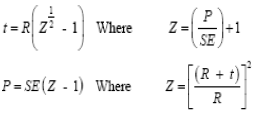Related Resources: pressure-vessel

### Thick Cylindrical Shells Pressure Vessel Equations and Calculator

ASME Pressure Vessel Design and Engineering

ASME SECTION VIII - Thick Cylindrical Shells Equations and Calculator:

For internal pressures higher than 3,000 psi, special considerations as specified in paragraph U-1 (d). As the ratio of t/R increases beyond 0.5, an accurate equation is required to determine the thickness. The formulae in ASME Appendix 1, Supplementary Design Formulas used for calculating thick wall and design pressure, are:

a) Longitudinal welds:
When, P > 0.385SE:Circumferential welds:
When, P > 1.25SEWhere:
t = Minimum Design Wall Thickness (in);
D = Tube Outside Diameter (in);
E = Welding Factor (1.0 for seamless pipe; 0.85 = for welded pipe);
Z = Dimensionless Factor;
C = Corrosion Allowance (0 for no corrosion; 0.0625 in. commonly used; 0.125 in. maximum);
S = Maximum Allowable Stress According to ASME Section II.